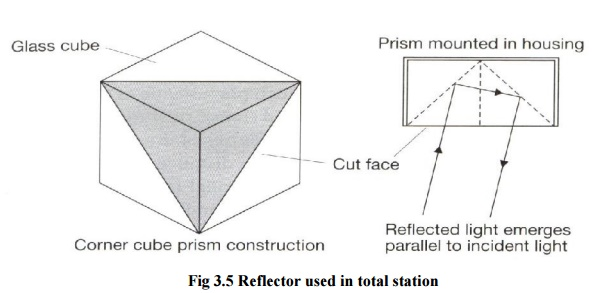Home | | Surveying | Classification of Total Stations

# Classification of Total Stations

Distances are measured using two methods: the phase shift method, and the pulsed laser method.

CLASSIFICATION OF TOTAL STATIONS

1       ELECTRO- OPTICAL SYSTEM

1.1DISTANCE MEASUREMENT

When a distance is measured with a total station, am electromagnetic wave or pulse is used for the measurement - this is propagated through the atmosphere from the instrument to reflector or target and back during the measurement.

Distances are measured using two methods: the phase shift method, and the pulsed laser method.

This technique uses continuous electromagnetic waves for distance measurement although these are complex in nature, electromagnetic waves can be represented in their simplest from as periodic waves.Fig 3.3 Sinusoidal wave motion

The wave completes a cycle when moving between identical points on the wave and the number of times in one second the wave completes the cycle is called the frequency of the wave. The speed of the wave is then used to estimate the distance.

2 LASER DISTANCE MEASUREMENT

In many total stations, distances are obtained by measuring the time taken for a pulse of laser radiation to travel from the instrument to a prism (or target) and back. As in the phase shift method, the pulses are derived an infrared or visible laser diode and they are transmitted through the telescope towards the remote end of the distance being measured, where they are reflected and returned to the instrument.

Since the velocity v of the pulses can be accurately determined, the distance D can be obtained using 2D = vt, where t is the time taken for a single pulse to travel from instrument - target - instrument.

This is also known as the timed-pulse or time-of-flight measurement technique.

The transit time t is measured using electronic signal processing techniques. Although only a single pulse is necessary to obtain a distance, the accuracy obtained would be poor. To improve this, a large number of pulses (typically

20,000 every second) are analysed during each measurement to give a more accurate distance.

The pulse laser method is a much simpler approach to distance measurement than the phase shift method, which was originally developed about 50 years ago.

SLOPE AND HORIZONTAL DISTANCES

Both the phase shift and pulsed laser methods will measure a slope distance L from the total station along the line of sight to a reflector or target. For most surveys the horizontal distance D is required as well as the vertical component V of the slope distance.

Horizontal distance D = L cos? = L sin z

Vertical distance = V = L sin? = L cos z

Where ? is the vertical angle and z is the is the zenith angle.   As   far   as   the   user   is

concerned, these calculations are seldom done because the total station will

either display D and V automatically or will dislplay L first and then D and V after pressing buttonsFig 3.4 Slope and Distance Measured

How accuracy of distance measurement is specified

All total stations have a linear accuracy quoted in the form

�(a mm + b ppm)

The constant a is independent of the length being measured and is made up of internal sources within the instrument that are normally beyond the control of the user. It is an estimate of the individual errors caused by such phenomena as unwanted phase shifts in electronic components, errors in phase and transit time measurements.

The systematic error b is proportional to the distance being measured, where 1 ppm (part per million) is equivalent to an additional error of 1mm for every kilometre measured.

Typical specifications for a total station vary from �(2mm + 2ppm) to �(5mm + 5 pmm).

For example: �(2mm + 2ppm), at 100m the error in distance measurement will be

�2mm but at 1.5km, the error will be �(2mm + [2mm/km * 1.5km]) = �5m  m

Reflectors used in distance measurement

Since the waves or pulses transmitted by a total station are either visible or infrared, a plane mirror could be used to reflect them. This would require a very accurate alignment of the mirror, because the transmitted wave or pulses have a narrow spread.

To get around this problem special mirror prisms are used as shown below.Fig 3.5 Reflector used in total station

Study Material, Lecturing Notes, Assignment, Reference, Wiki description explanation, brief detail
Civil Surveying : Total Station : Classification of Total Stations |